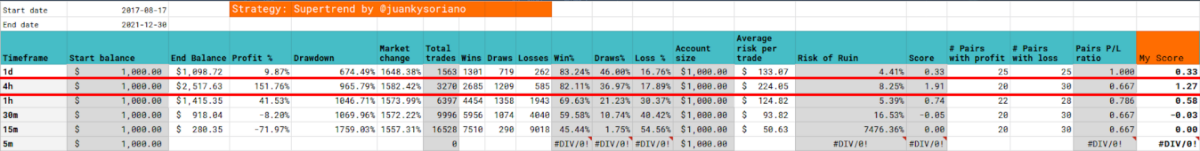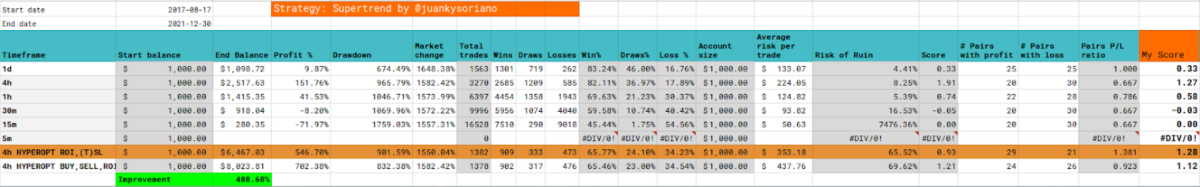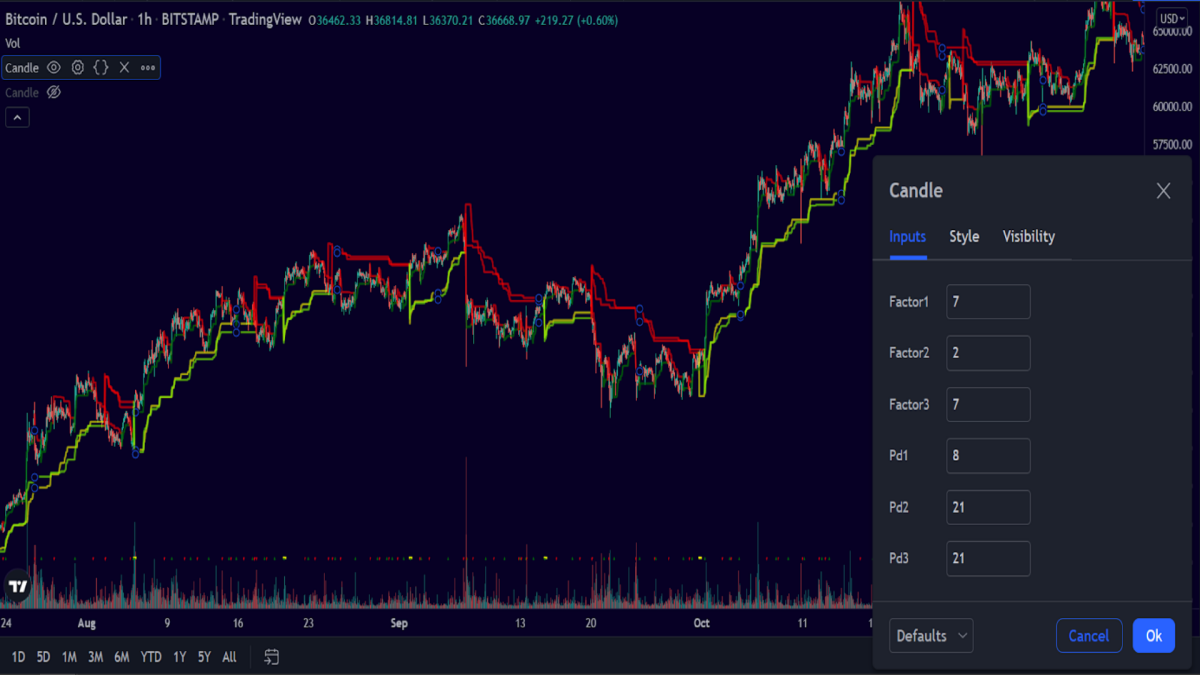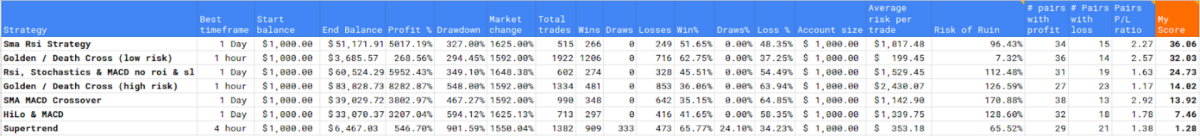# Supertrend (3x) strategy - Is this strategy profitable or not?

### Intro

In this blog series I will be searching for the best trading strategy for cryptocurrency trading with a trading bot. These posts are complementary to the video's I make on Youtube, which you can watch below.

The trading bot I use is the Freqtrade (https://www.freqtrade.io/en/latest/) trading bot which not only has good options for bot trading, but also is excellent in backtesting and optimization of trading parameters. Therefore I will use this program to not only trade automatically, but also look for the best strategies and setups.

All code that is specifically written for this strategy or for hyperparameter optimisation is available at the bottom of this post.

If you appreciate the effort I take to create this strategy or optimisation code, then please consider a tip for this post!

### The backtesting setup

If you want to know more on how I do these backtests like: which pairs I use, which timeframes and which periods I test, then see this blog post to know more about the approach I use: https://www.publish0x.com/dutchcryptodad/is-it-profitable-or-not-the-setup-and-approach-xppgjoj

### Strategy

The strategy makes use of three supertrend lines and the strategy is very simple.

• When all three supertrend lines have a buy signal (all three are green). Then the bot will buy.
• And when all supertrend indicators are red, then a sell signal occurs and the bot will sell.

This description is also written down in the code of the strategy file.

### Initial backtest results• The best timeframe is the 4 hour timeframe.
• It has the larges profit of 151 percent which could have hypothetically turned 1000 dollar into 2517 dollar over the complete backtesting period
• The drawdown is 956 percent and the possible risk of ruin is 8.25 percent.

The reason why this risk of ruin is so low is that there are a lot more winning trades than losing trades. You can also see that this strategy also counts a large amount of draws. You can say that this is a good sign because the trade did not turn into a losing position. However, because you have to pay trading fees to the exchange I consider these trades also as losers. It might technically not be completely right and you may have your own opinion about this.

And a final thing I do not really like is the amount of drawdown that this strategy has. If there was a way to reduce this drawdown then there might be better results to get.

### Hyperparameter optimisation

The author of the code did a good job by already providing the buy and sell spaces and preprocess the supertend indicator to use for hyperopt. I had to modify the strategy a little bit to enable hyperopt. But that was no big deal.

I had to split the hyperopt session into two parts to get some output of this analysis phase. So I first did a hyperopt session to calculate the best ROI, Stoploss and trailing stoploss on the default settings of the Supertrend indicators. And after that I did a second hyperopt session to see which buy and sell parameters would be advised.

When you stick to the default supertrend indicator parameters, and only look for better roi, stoploss and trailing stoploss settings, then you indeed get better results in comparison with the original settings. But there not spectacular.

Changing the supertrend indicators after that gave you even better results so this proves to be even better. But the winrate gets slightly worse and also these settings do not work as good on all the pairs. So with my score calculation only the roi and stoploss change gets slightly better results.

### Conclusions• Hyperopt under special circumstances
• Only 5 pairs used (BTC, LTC, XRP, ADA, BNB)
• Only 100 Epochs
• Split hyperopt analysis in two parts (ROI, SL & TSL) + (BUY & SELL)

As you can see, two supertrends are almost identical to each other.

The Supertrend one and three look very similar. The only supertrend that is out of line here is the second supertrend indicator.

The Supertrend 1`F1(1)P1(21) and Supertrend 2 F2(1)P2(7) have different numbers but have almost the same supertrend line. The Supertrend 3 Is a totally different line and I wonder if using only two supertrends will do a better job than using three supertrend.### Strategy League

And as you can see, the supertrend performs the worst of all strategies. And actually this surprises me a little bit.

Maybe this is because it uses a lower timeframe and thus do more trades. Or maybe it because this particular strategy uses two sets of three indicators for producing buy and sell signals. After watching the indicator with the hyperopted parameters I think this indicator should get a second chance and be used in a much less complicated way.### The strategy / Hyperopt code

``````"""
Supertrend strategy:
* Description: Generate a 3 supertrend indicators for 'buy' strategies & 3 supertrend indicators for 'sell' strategies
Sells if the 3 'sell' indicators are 'down'
* Author: @juankysoriano (Juan Carlos Soriano)
* github: https://github.com/juankysoriano/

*** NOTE: This Supertrend strategy is just one of many possible strategies using `Supertrend` as indicator. It should on any case used at your own risk.
It comes with at least a couple of caveats:
2. The implementation for `supertrend` on this strategy is not validated; meaning this that is not proven to match the results by the paper where it was originally introduced or any other trusted academic resources
"""

import logging
from numpy.lib import math
from pandas import DataFrame
import talib.abstract as ta
import numpy as np

class Supertrend(IStrategy):
# Buy params, Sell params, ROI, Stoploss and Trailing Stop are values generated by 'freqtrade hyperopt --strategy Supertrend --hyperopt-loss ShortTradeDurHyperOptLoss --timerange=20210101- --timeframe=1h --spaces all'
# It's encourage you find the values that better suites your needs and risk management strategies

}

# Sell hyperspace params:
sell_params = {
"sell_m1": 1,
"sell_m2": 3,
"sell_m3": 6,
"sell_p1": 16,
"sell_p2": 18,
"sell_p3": 18,
}

# ROI table:
minimal_roi = {
"0": 0.087,
"372": 0.058,
"861": 0.029,
"2221": 0
}

# Stoploss:
stoploss = -0.265

# Trailing stop:
trailing_stop = True
trailing_stop_positive = 0.05
trailing_stop_positive_offset = 0.144
trailing_only_offset_is_reached = False

timeframe = '1h'

startup_candle_count = 18

sell_m1 = IntParameter(1, 7, default=4)
sell_m2 = IntParameter(1, 7, default=4)
sell_m3 = IntParameter(1, 7, default=4)
sell_p1 = IntParameter(7, 21, default=14)
sell_p2 = IntParameter(7, 21, default=14)
sell_p3 = IntParameter(7, 21, default=14)

def populate_indicators(self, dataframe: DataFrame, metadata: dict) -> DataFrame:

for multiplier in self.sell_m1.range:
for period in self.sell_p1.range:
dataframe[f'supertrend_1_sell_{multiplier}_{period}'] = self.supertrend(dataframe, multiplier, period)['STX']

for multiplier in self.sell_m2.range:
for period in self.sell_p2.range:
dataframe[f'supertrend_2_sell_{multiplier}_{period}'] = self.supertrend(dataframe, multiplier, period)['STX']

for multiplier in self.sell_m3.range:
for period in self.sell_p3.range:
dataframe[f'supertrend_3_sell_{multiplier}_{period}'] = self.supertrend(dataframe, multiplier, period)['STX']

return dataframe

dataframe.loc[
(
(dataframe['volume'] > 0) # There is at least some trading volume
),

return dataframe

def populate_sell_trend(self, dataframe: DataFrame, metadata: dict) -> DataFrame:
dataframe.loc[
(
(dataframe[f'supertrend_1_sell_{self.sell_m1.value}_{self.sell_p1.value}'] == 'down') &
(dataframe[f'supertrend_2_sell_{self.sell_m2.value}_{self.sell_p2.value}'] == 'down') &
(dataframe[f'supertrend_3_sell_{self.sell_m3.value}_{self.sell_p3.value}'] == 'down') & # The three indicators are 'down' for the current candle
(dataframe['volume'] > 0) # There is at least some trading volume
),
'sell'] = 1

return dataframe

"""
"""
def supertrend(self, dataframe: DataFrame, multiplier, period):
df = dataframe.copy()

df['TR'] = ta.TRANGE(df)
df['ATR'] = ta.SMA(df['TR'], period)

st = 'ST_' + str(period) + '_' + str(multiplier)
stx = 'STX_' + str(period) + '_' + str(multiplier)

# Compute basic upper and lower bands
df['basic_ub'] = (df['high'] + df['low']) / 2 + multiplier * df['ATR']
df['basic_lb'] = (df['high'] + df['low']) / 2 - multiplier * df['ATR']

# Compute final upper and lower bands
df['final_ub'] = 0.00
df['final_lb'] = 0.00
for i in range(period, len(df)):
df['final_ub'].iat[i] = df['basic_ub'].iat[i] if df['basic_ub'].iat[i] < df['final_ub'].iat[i - 1] or df['close'].iat[i - 1] > df['final_ub'].iat[i - 1] else df['final_ub'].iat[i - 1]
df['final_lb'].iat[i] = df['basic_lb'].iat[i] if df['basic_lb'].iat[i] > df['final_lb'].iat[i - 1] or df['close'].iat[i - 1] < df['final_lb'].iat[i - 1] else df['final_lb'].iat[i - 1]

# Set the Supertrend value
df[st] = 0.00
for i in range(period, len(df)):
df[st].iat[i] = df['final_ub'].iat[i] if df[st].iat[i - 1] == df['final_ub'].iat[i - 1] and df['close'].iat[i] <= df['final_ub'].iat[i] else \
df['final_lb'].iat[i] if df[st].iat[i - 1] == df['final_ub'].iat[i - 1] and df['close'].iat[i] >  df['final_ub'].iat[i] else \
df['final_lb'].iat[i] if df[st].iat[i - 1] == df['final_lb'].iat[i - 1] and df['close'].iat[i] >= df['final_lb'].iat[i] else \
df['final_ub'].iat[i] if df[st].iat[i - 1] == df['final_lb'].iat[i - 1] and df['close'].iat[i] <  df['final_lb'].iat[i] else 0.00
# Mark the trend direction up/down
df[stx] = np.where((df[st] > 0.00), np.where((df['close'] < df[st]), 'down',  'up'), np.NaN)

# Remove basic and final bands from the columns
df.drop(['basic_ub', 'basic_lb', 'final_ub', 'final_lb'], inplace=True, axis=1)

df.fillna(0, inplace=True)

return DataFrame(index=df.index, data={
'ST' : df[st],
'STX' : df[stx]
})
``````

### The hyperopt json results

``````{
"strategy_name": "SupertrendHopt",
"params": {
"roi": {
"0": 0.529,
"1829": 0.193,
"4694": 0.099,
"9878": 0
},
"stoploss": {
"stoploss": -0.298
},
"trailing": {
"trailing_stop": true,
"trailing_stop_positive": 0.194,
"trailing_stop_positive_offset": 0.28,
"trailing_only_offset_is_reached": true
},
},
"sell": {
"sell_m1": 1,
"sell_m2": 1,
"sell_m3": 6,
"sell_p1": 21,
"sell_p2": 7,
"sell_p3": 15
},
"protection": {}
},
"ft_stratparam_v": 1,
"export_time": "2022-01-25 09:59:54.110863+00:00"
}

``````I'm just a regular Dutch dad with a passion for crypto, trading, technology and learning. This channel is my personal journey into the world of Crypto, blockchain, programming, trading bots, trading strategies, NFT's, Defi and many things more.